Home > CCA2 > Chapter 9 > Lesson 9.3.2 > Problem9-95

9-95.
1. Rewrite each equation in graphing form and identify the key points. Homework Help ✎

1. y =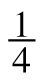x2 +x +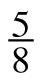2. y =x2 + 5x + 41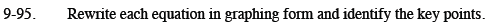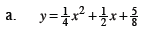Multiply both sides by 4.

$4y=x^2+2x+\frac{5}{2}$

Move 'c' to the left side.

$4y-\frac{5}{2}=x^2+2x$

Complete the square.

$4y-\frac{3}{2}=x^2+2x+1$

Factor both sides.

$4\left(y-\frac{3}{8}\right)=\left(x+1\right)^2$

Rewrite in y = form.

$y=\frac{1}{4}(x+1)^2+\frac{3}{8}$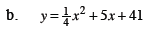Follow the steps outlined in part (a).